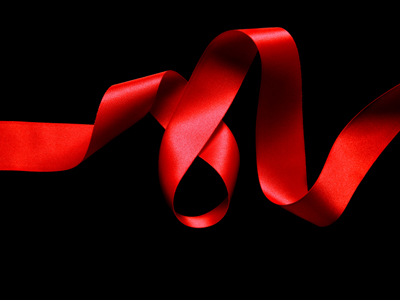It takes 30cm of ribbon to make a bow. 6 bows can be made from a 2m long ribbon.

# Solving Problems 4

This Math quiz is called 'Solving Problems 4' and it has been written by teachers to help you if you are studying the subject at elementary school. Playing educational quizzes is an enjoyable way to learn if you are in the 3rd, 4th or 5th grade - aged 8 to 11.

It costs only \$12.50 per month to play this quiz and over 3,500 others that help you with your school work. You can subscribe on the page at Join Us

Solving problems requires either multiplication, division, addition or subtraction, and possibly all of them! In real life you'll come across problems involving weights and measures - for example, how much money something costs in dollars and cents or how much it weighs in grams and kilograms. But not all problems are about measures, some are different, like this one - If your doctor has told you to take a Vitamin C tablet every day for 3 months, how many tablets will you need to buy?

See how much you have learned in your lessons by playing this quiz about solving problems involving different measures.

1.
There were three jugs; one held 0.75 liters, another held 400ml and the other held 350ml. How much did the jugs hold altogether?
1 liter
1.25 liters
1.5 liters
1.75 liters
To work out the answer you have to add the three amounts together. 0.75 liters is the same as 750ml so 750 + 400 + 350 = 1,500ml which is the same as 1.5 liters
2.
Dad is 1.83m tall. The ceiling is 28cm higher than Dad. How high is the ceiling?
2m
2.01m
2.10m
2.11m
There are 100cm in a meter so Dad is 183cm tall. 183 + 28 = 211 so the ceiling is 211cm tall. 211cm is the same as 2.11 meters
3.
How many kilograms of sugar must be added to 2.34kg to make 6kg?
2.66
2.76
3.66
3.76
100 - 34 = 66 so we know that the answer will end in .66. If we add 0.66 to 2.34 we get 3 so we still need another 3kg
4.
I think of a number and multiply it by 15. I subtract 180. The answer is 300. What was my number?
20
22
28
32
This type of question needs to go backwards. The last step was to subtract 180 so add this back 300 + 180 = 480. To get here we had to multiply by 15 so we divide by 15 to get back to our original number
5.
A car uses 1.2 liters of fuel for every 5 miles. The car has 20 liters of fuel in the tank. How much fuel will be left after the car has traveled 60 miles?
4.6 liters
5.6 liters
6.6 liters
14.6 liters
To work this one out first divide 60 by 5 which is 12. Then Multiply 12 by 1.2 which is 14.4 so the car will use up 14.4 liters of fuel if it travels 60 miles. 14.4 liters subtracted from 20 liters leaves 5.6 liters still in the tank
6.
Lamb takes 50 minutes per kg to cook plus 50 minutes. How long does it take for a 2.5kg piece of lamb to cook?
1 hour 15 minutes
1 hour and 55 minutes
165 minutes
2 hours and 55 minutes
To calculate this 50 minutes x 2.5 = 125 minutes. Add another 50 minutes gives 175 minutes or 2 hours and 55 minutes
7.
A race lasting 3 hours and 40 minutes ended at 16:50. What time did the race start?
13:10
13:20
14:10
14:20
To work this out first take 40 minutes from 50 leaving 10. Then take 3 hours from 16 leaving 13
8.
Martin traveled 31.2km by car, 5.8km by bus and 0.23km on foot. How far did he travel altogether?
35.32km
37.23km
37.32km
38.32km
Adding the numbers after the decimal points will help here. 0.2 + 0.8 = 1.0 so we know that the answer will end in .23
9.
At the movie theater there are 42 seats in each row and 32 rows. How many seats altogether?
1,244
1,344
1,354
1,534
If there are 42 seats in every row then we multiply 42 by 32 to work out the answer
10.
It takes 30cm of ribbon to make a bow. How many bows can be made from a 2m long ribbon?
5
6
7
8
200 ÷ 30 = 6 remainder 20cm. As 20cm is not long enough to make a bow, we can only make 6 bows
Author:  Amanda Swift